# Boolean algebra

Also found in: Dictionary, Thesaurus, Medical, Wikipedia.

## Boolean algebra

(bo͞o`lēən), an abstract mathematical system primarily used in computer science and in expressing the relationships between setsset,
in mathematics, collection of entities, called elements of the set, that may be real objects or conceptual entities. Set theory not only is involved in many areas of mathematics but has important applications in other fields as well, e.g.
..... Click the link for more information.
(groups of objects or concepts). The notational system was developed by the English mathematician George BooleBoole, George,
1815–64, English mathematician and logician. He became professor at Queen's College, Cork, in 1849. Boole wrote An Investigation of the Laws of Thought (1854) and works on calculus and differential equations.
..... Click the link for more information.
c.1850 to permit an algebraic manipulation of logical statements. Such manipulation can demonstrate whether or not a statement is true and show how a complicated statement can be rephrased in a simpler, more convenient form without changing its meaning. In his 1881 treatise, Symbolic Logic, the English logician and mathematician John Venn interpreted Boole's work and introduced a new method of diagramming Boole's notation; this was later refined by the English mathematician Charles Dodgson (better known as Lewis CarrollCarroll, Lewis,
pseud. of Charles Lutwidge Dodgson,
1832–98, English writer, mathematician, and amateur photographer, b. near Daresbury, Cheshire (now in Halton).
..... Click the link for more information.
—this method is now know as the Venn diagram. When used in set theory, Boolean notation can demonstrate the relationship between groups, indicating what is in each set alone, what is jointly contained in both, and what is contained in neither. Boolean algebra is of significance in the study of information theory, the theory of probability, and the geometry of sets. The expression of electrical networks in Boolean notation has aided the development of switching theory and the design of computers.
The Columbia Electronic Encyclopedia™ Copyright © 2013, Columbia University Press. Licensed from Columbia University Press. All rights reserved. www.cc.columbia.edu/cu/cup/

## Boolean algebra

[′bü·lē·ən ′al·jə·brə]
(mathematics)
An algebraic system with two binary operations and one unary operation important in representing a two-valued logic.
McGraw-Hill Dictionary of Scientific & Technical Terms, 6E, Copyright © 2003 by The McGraw-Hill Companies, Inc.

## Boolean algebra

a system of symbolic logic devised by George Boole to codify logical operations. It is used in computers
Collins Discovery Encyclopedia, 1st edition © HarperCollins Publishers 2005

## Boolean algebra

(mathematics, logic)
(After the logician George Boole)

1. Commonly, and especially in computer science and digital electronics, this term is used to mean two-valued logic.

2. This is in stark contrast with the definition used by pure mathematicians who in the 1960s introduced "Boolean-valued models" into logic precisely because a "Boolean-valued model" is an interpretation of a theory that allows more than two possible truth values!

Strangely, a Boolean algebra (in the mathematical sense) is not strictly an algebra, but is in fact a lattice. A Boolean algebra is sometimes defined as a "complemented distributive lattice".

Boole's work which inspired the mathematical definition concerned algebras of sets, involving the operations of intersection, union and complement on sets. Such algebras obey the following identities where the operators ^, V, - and constants 1 and 0 can be thought of either as set intersection, union, complement, universal, empty; or as two-valued logic AND, OR, NOT, TRUE, FALSE; or any other conforming system.

a ^ b = b ^ a a V b = b V a (commutative laws) (a ^ b) ^ c = a ^ (b ^ c) (a V b) V c = a V (b V c) (associative laws) a ^ (b V c) = (a ^ b) V (a ^ c) a V (b ^ c) = (a V b) ^ (a V c) (distributive laws) a ^ a = a a V a = a (idempotence laws) --a = a -(a ^ b) = (-a) V (-b) -(a V b) = (-a) ^ (-b) (de Morgan's laws) a ^ -a = 0 a V -a = 1 a ^ 1 = a a V 0 = a a ^ 0 = 0 a V 1 = 1 -1 = 0 -0 = 1

There are several common alternative notations for the "-" or logical complement operator.

If a and b are elements of a Boolean algebra, we define a <= b to mean that a ^ b = a, or equivalently a V b = b. Thus, for example, if ^, V and - denote set intersection, union and complement then <= is the inclusive subset relation. The relation <= is a partial ordering, though it is not necessarily a linear ordering since some Boolean algebras contain incomparable values.

Note that these laws only refer explicitly to the two distinguished constants 1 and 0 (sometimes written as LaTeX \top and \bot), and in two-valued logic there are no others, but according to the more general mathematical definition, in some systems variables a, b and c may take on other values as well.
This article is provided by FOLDOC - Free Online Dictionary of Computing (foldoc.org)

## Boolean logic

The "mathematics of logic," developed by English mathematician George Boole in the mid-19th century. Just as add, subtract, multiply and divide are the primary operations of arithmetic, AND, OR and NOT are the primary logical operators of Boolean logic and building blocks of every digital circuit. NAND (not AND), NOR (not OR) and XOR (exclusive OR) are variations of AND, OR and NOT (see NAND, NOR and XOR). See Boolean search, binary, logic gate and Bebop to the Boolean Boogie.

Decimal vs. Binary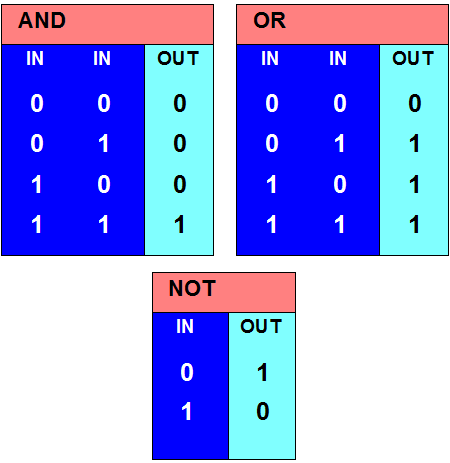Decimal arithmetic holds 10 values (0 to 9) in each digit position. In binary, there are only two (0 and 1). Note below the four possible result and carry bits when adding two binary digits (bits) together.

AND, OR and NOT Gates
Transistors wired in series and parallel patterns make up these "gates," which accept inputs of 0 (no pulse) or 1 (a pulse) and generate outputs of 0 or 1. While AND requires that both inputs are 1 to generate an output of 1, OR only needs one input to be 1. NOT reverses the input. For a diagram of these actions, see Boolean gates.

Decimal vs. Binary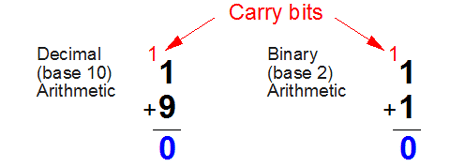Decimal arithmetic holds 10 values (0 to 9) in each digit position. In binary, there are only two (0 and 1). Note below the four possible result and carry bits when adding two binary digits (bits) together.

Add a One and Zero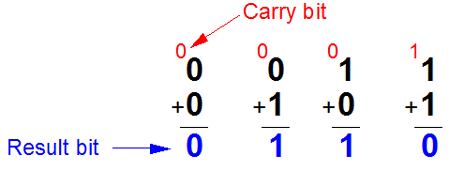Trace the red 1 (pulse, current) and white 0 (no pulse, no current) through the gates and notice their outputs. This half-adder circuit is in every CPU.

Add a One and Zero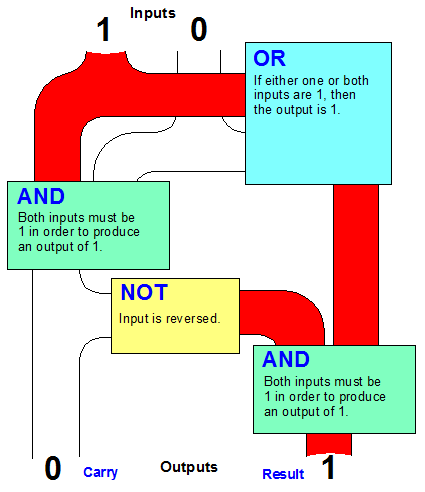Trace the red 1 (pulse, current) and white 0 (no pulse, no current) through the gates and notice their outputs. This half-adder circuit is in every CPU.

Trace the Flow YourselfChoose any two binary digits as input and trace them through this half-adder circuit (0 is no current; 1 is current). Watch how the output is generated.

Trace the Flow Yourself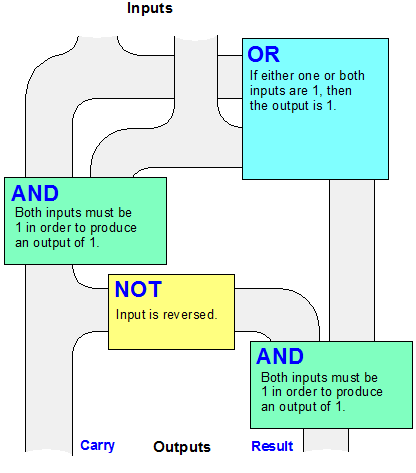Choose any two binary digits as input and trace them through this half-adder circuit (0 is no current; 1 is current). Watch how the output is generated.

Patterns of Boolean Logic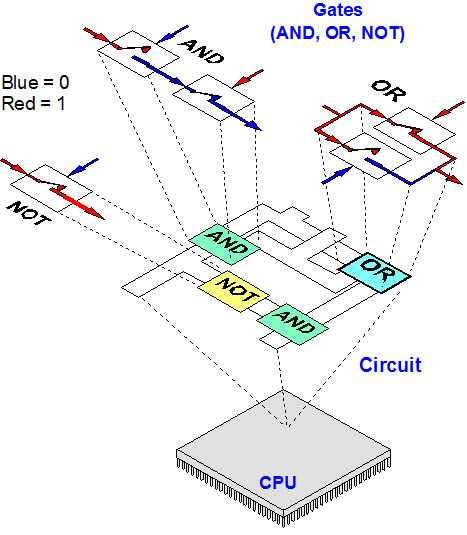Transistors (depicted here as mechanical switches) make up gates. Gates make up circuits, and circuits make up every digital device. There are millions and billions of transistors in modern chips, and they fit in an area smaller than a postage stamp. For more details, see Boolean gates. See transistor and chip.
Copyright © 1981-2019 by The Computer Language Company Inc. All Rights reserved. THIS DEFINITION IS FOR PERSONAL USE ONLY. All other reproduction is strictly prohibited without permission from the publisher.
References in periodicals archive ?
Interpolative Boolean algebra (IBA) is the Boolean consistent [0,1]-valued generalization of finite (atomic) Boolean algebra (BA) [30, 39].
"[product sum]" indicates XOR Boolean algebra operation.
In this section we study the Stanley-Reisner ring of the Boolean algebra. The Boolean algebra [B.sub.n] is the ranked poset of all subsets of [n] := {1,2, ..., n} ordered by inclusion, with minimum element [phi] and maximum element [n].
Let L be a complete Boolean algebra. Then k-regularity [??] [k.sub.*]-regularity and k'-regularity [??] [k.sub.'*]-regularity.
(i) (2, [conjunction], [disjunction], [(-).sup.~]) is a Boolean algebra. So every Boolean algebra is a Pre [A.sup.*]- algebra
Theorem 2.14 The family F is an effective Boolean algebra, i.e., given two subsets X, Y [subset or equal to] [Z.sub.n] specified as in Definition 2.12, there exists a procedure that computes the specification of the subsets X [union] Y, X [union] Y and [Z.sub.n] \ X.
The purpose of this article is not to discourage use of these time honored research strategies, but to suggest the inclusion of a method using crisp sets and Boolean algebra as a research strategy, and to illustrate this method by example, and to discuss how this method can be of value to student affairs researchers.
The first two chapters introduce readers to basic logic circuits and Boolean algebra. Even if you are an expert logic designer, but without VHDL experience, read these chapters thoroughly because they describe basic concepts.
It's designed as a textbook, so college-level students and practicing engineers will find it most handy--but it covers all the basics, from Boolean algebra and logic gates to Karnaugh maps.
The algorithm combines complex network mathematics and Boolean algebra to define regions on a batch of CNTs.
Many users of web are not well trained in Boolean algebra; "the problem of learning the correct interpretations of Boolean operators and their rules of precedence" .

Site: Follow: Share:
Open / Close